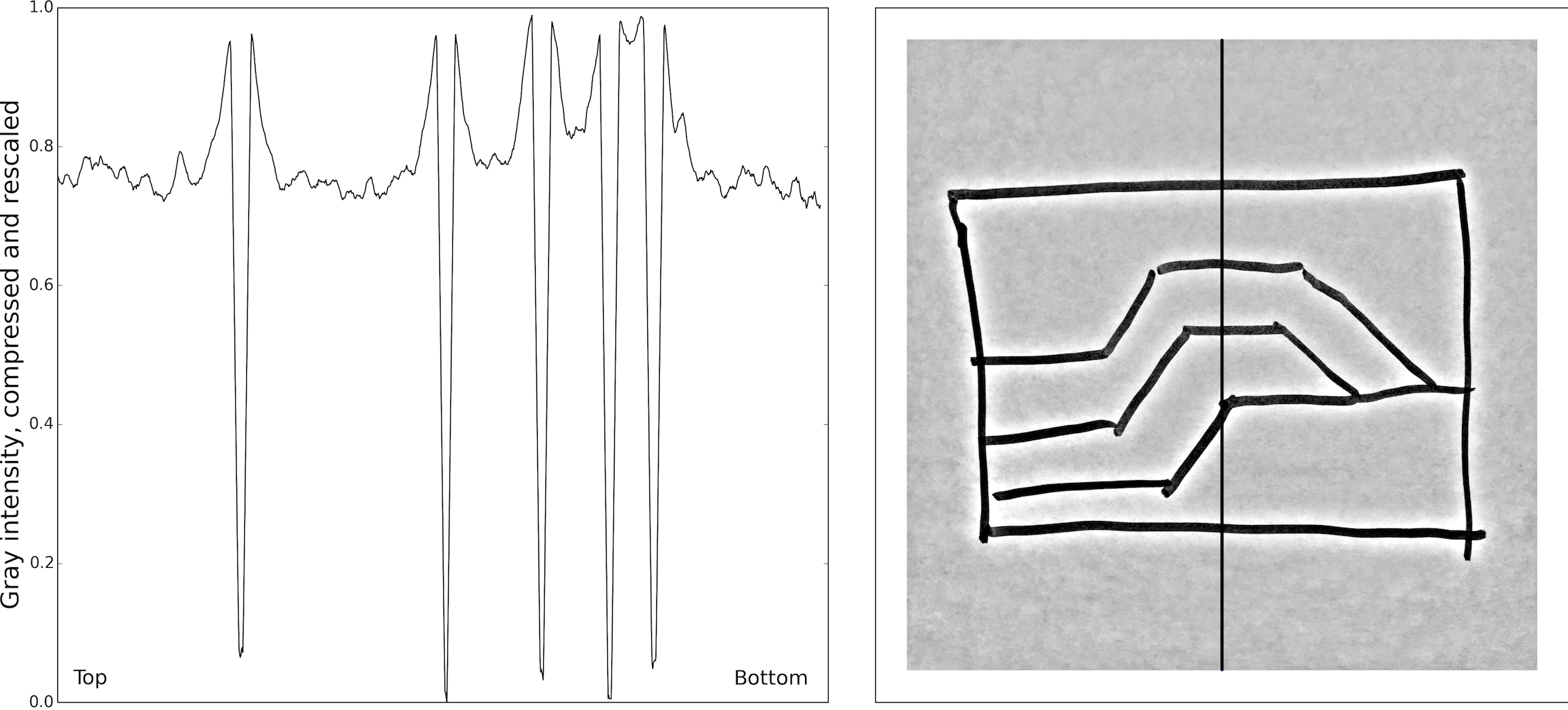# sketch2model – sketch image enhancements

This is the second post of in a series of collaborative articles about sketch2model, a project from the 2015 Calgary Geoscience Hackathon organized by Agile Geoscience.

The first post was written by Elwyn Galloway and published on both his Scibbatical blog and here on MyCarta. In that article Elwyn mentioned the need for an adaptive image conditioning workflow for binarization of photos with geological sketches in images. Binarization is the process of converting a natural image to a binary image (check this simple but awesome interactive demonstration of binarization), which in our case is necessary to separate the sketch from the background.

The following is a demonstration of the preliminary image processing operations applied to the input photo when sketch2model is run. The full code listing of this demonstration is available as a Jupyter notebook on GitHub. Also in GitHub you can find a Jupyter Notebook with the fully documented version of sketch2model.

First we import one of the photos with sketches and convert it to a grayscale image.

```im = io.imread('paper_breaks.png')
im = color.rgb2gray(im[0:-1:2,0:-1:2])```Next we enhance the grayscale image with a couple of cascaded processes. But before we do that, let’s graph the intensity values across the image to understand the degree of contrast between sketch edges and background, which ultimately will determine our success in separating them from it. We show this in the figure below, on the left, for one column of pixels (y direction). The black line across the input image on the right shows the location of the column selected. It is fairly obvious from the plot on the left that the intensity of the background is not uniform, due to variable light conditions when the photo was taken, and towards the right (e.g. bottom of the photo) it gets closer to that of the edges. In some images it might even become less than the intensity of the edge. This highlights the need for (preemptively) applying the enhancements illustrated in the remainder of the post.The first enhancement is called compressor, or limiter. I read many years ago that it is used in electronics to find hard edges in data: the idea is to square each element in the data (image, or other type of data), smooth the result (enough to remove high frequency variations but not so much as to eliminate variability), take the square root, and finally divide each element in the input by the square root result.

I experimented with this method (at the time using Matlab and its Image Processing Toolbox) using the same gravity dataset from my 2015 geophysical tutorial on The Leading Edge (see the post Mapping and validating geophysical lineaments with Python). An example of one such experiments is shown in the figure below where: the top left map is the Bouguer data; the centre top map is the squared data; the top right is the result of a Gaussian blur; the bottom left the result of square root, and centre right is the final output, where the hardest edges in the original data have been enhanced.The most important parameter in this process is the choice of the smoothing or blur; using a Gaussian kernel of different size more subtle edges are enhanced, as seen in the bottom right map (these are perhaps acquisition-related gridding artifacts).

In our sketch2model implementation the size of the Gaussian kernel is hardcoded; it was chosen following trial and error on multiple photos of sketches and yielded optimal results in the greatest majority of them. We were planning to have the kernel size depend on the size of the input image, but left the implementation to our ‘future work’ list.’

Here’s the compressor code from sketch2model:

```# compressor or limiter (electronics): find hard edges in data with long
# wavelength variations in amplitude
# step 1: square each element in the image to obtain the power function
sqr = im**2
# step 2: gaussian of squared image
flt2 = sp.ndimage.filters.gaussian_filter(sqr,21)
# step 3: divide the intensity of each original pixel by the square root
# of the smoothed square
cmprs= im/(np.sqrt(flt2))```

and a plot of the result (same column of pixels as in the previous one):From the plot above we see that now the background intensity is uniform and the contrast has been improved. We can maximize it with contrast stretching, as below:

```# contrast stretching
p2, p98 = np.percentile(cmprs, (2, 98))
rescale = exposure.rescale_intensity(cmprs, in_range=(p2, p98))
```We now have ideal contrast between edges and background, and can get a binary image with the desired sketch edges using a scalar threshold:

```# binarize image with scalar threshold
binary = ~(color.rgb2gray(rescale) > 0.5)```Bingo!

## 7 thoughts on “sketch2model – sketch image enhancements”

1.Penny on said:

Hmm enhancement techniques, for seismic data, likely have connections to “PowerPoint” picture and color correction options as “enhancements”. Likely the techniques behind the “buttons” for “sharpness /softness ” or “contrast” , and color “saturation” or hue have math behind the buttons. Anyone ever found where to read the descriptions of the math behind the “photo” equivalent techniques.?

•Matteo on said:

Hello Penny.

Good question. The answer is both yes and no. Yes, the contrast stretching is similar to what you would do in Photoshop (not sure about PowePoint, I have not checked). No, in that the compressor is a more sophisticated technique, where you manipulate the image (square it), apply a smoothing blur (Gaussian), take the root, then subtract from the original. You could probably achieve this in Photoshop with a combination of operations, but I don’t think there’s a ready operation.

My favorite all-around resource on image manipulation is the Imagemagik usage guide: http://www.imagemagick.org/Usage/

2. Pingback: Mild or Wild – scibbatical